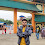# HackerRank Validating and Parsing Email Addresses solution in python

In this Validating and Parsing Email Addresses problem, you have Given N pairs of names and email addresses as input, print each name and email address pair having a valid email address on a new line.

## Problem solution in Python 2 programming.

```# Enter your code here. Read input from STDIN. Print output to STDOUT
import re
N = input()
for i in xrange(N):
s = raw_input()
a = re.search(r'<[a-zA-Z][\w\.-]*@[a-zA-Z]*\.[a-zA-Z]{1,3}>', s)
if bool(a):
print(s)```

## Problem solution in Python 3 programming.

```# Enter your code here. Read input from STDIN. Print output to STDOUT
import re
n = int(input())
for _ in range(n):
x, y = input().split(' ')
m = re.match(r'<[A-Za-z](\w|-|\.|_)+@[A-Za-z]+\.[A-Za-z]{1,3}>', y)
if m:
print(x,y)```

### Problem solution in pypy programming.

```import email.utils
import re
n = int(raw_input())
for i in range(n):
if re.match(r'[a-z][\w.-]+@[a-z]+\.[a-z]{1,3}\$', p, re.I):

### Problem solution in pypy3 programming.

```# Enter your code here. Read input from STDIN. Print output to STDOUT

import re
import email.utils
n = int(input().strip())
temp =[]
a = re.compile(r'<[a-z0-9][\w._-]+@[a-z]+\.[a-z]{1,3}>', re.I)
for _ in range(n):
temp.append(input().strip())
for i, x in enumerate(temp):
v =  a.search(x)
if v:
print(temp[i])```

1.1.